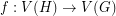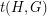Importance: High ✭✭✭
 Author(s): Sidorenko, A.
 Subject: Graph Theory
 Keywords: density problems extremal combinatorics homomorphism
 Posted by: Jon Noel on: October 10th, 2019
Conjecture   For any bipartite graphand graph, the number of homomorphisms fromtois at least.

A homomorphism from a graphto a graphis a mappingwhich preserves edges. Given graphsand, the homomorphism density ofin, denoted, is the probability that a random functionis a homomorphism. That is,In this language, Sidorenko's Conjecture says that, ifis bipartite, then every graphsatisfiesThere are lots of results on Sidorenko's Conjecture; rather than listing them all here, we encourage the reader to see the references of the recent paper [CL].

## Bibliography

[CL] David Conlon and Joonkyung Lee: Sidorenko's conjecture for blow-ups, submitted.

* indicates original appearance(s) of problem.